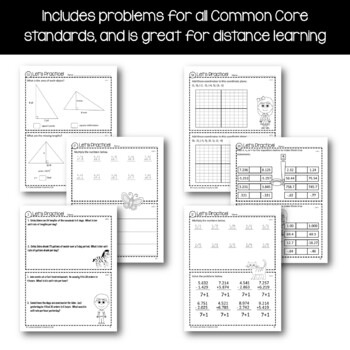# Homework for Sixth Grade Math - Answer Keys | Math Facts Fluency

Rated 5 out of 5, based on 22 reviews
22 Ratings;
6th - 8th, Homeschool
Subjects
Resource Type
Standards
Formats Included
• PDF
• Internet Activities
Pages
91 pages
\$12.00
\$12.00
Report this resource to TPT
Compatible with Digital Devices
The Teacher-Author has indicated that this resource can be used for device-based learning.

#### What educators are saying

My students enjoyed this resource. It was easy to access and fun to work on! There was a variety of activities to engage in and was really interactive.

### Description

Homework for Sixth Grade Math - Answer Keys Included! Includes 71 printables that you can give to your students throughout the school year to practice their skills according to the Math Common Core State Standards for the sixth grade. Each worksheet includes problems that will help your students work on their skills in mathematics. The Common Core standard or standards are clearly written on each section of the printable. Great for distance learning!

The worksheets can be used in the following ways:

• As take-home homework assignments
• As morning work or other in-class assignments
• As an introduction to specific Common Core skills
• As a daily review of Common Core skills learned
• As end-of-year review worksheets

All Common Core State Standards for 6th Grade Mathematics are covered in this packet. Answers are provided!

Find my other homework printables here:

All artwork is original and created by myself.

Thank you for visiting my store,

Yvonne Crawford

Total Pages
91 pages
Included
Teaching Duration
1 Year
Report this resource to TPT
Reported resources will be reviewed by our team. Report this resource to let us know if this resource violates TPT’s content guidelines.

### Standards

to see state-specific standards (only available in the US).
Find the area of right triangles, other triangles, special quadrilaterals, and polygons by composing into rectangles or decomposing into triangles and other shapes; apply these techniques in the context of solving real-world and mathematical problems.
Find the volume of a right rectangular prism with fractional edge lengths by packing it with unit cubes of the appropriate unit fraction edge lengths, and show that the volume is the same as would be found by multiplying the edge lengths of the prism. Apply the formulas 𝘝 = 𝘭 𝘸 𝘩 and 𝘝 = 𝘣 𝘩 to find volumes of right rectangular prisms with fractional edge lengths in the context of solving real-world and mathematical problems.
Draw polygons in the coordinate plane given coordinates for the vertices; use coordinates to find the length of a side joining points with the same first coordinate or the same second coordinate. Apply these techniques in the context of solving real-world and mathematical problems.
Represent three-dimensional figures using nets made up of rectangles and triangles, and use the nets to find the surface area of these figures. Apply these techniques in the context of solving real-world and mathematical problems.
Recognize a statistical question as one that anticipates variability in the data related to the question and accounts for it in the answers. For example, “How old am I?” is not a statistical question, but “How old are the students in my school?” is a statistical question because one anticipates variability in students’ ages.